Properties
 Home | Definitions | Interpretations | Applications | Gambling | Software | Books

Basic properties of the probability function

(P1)  For any, we have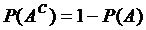(P2)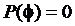(P3)  For any,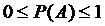(P4)  For any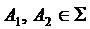with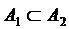, we have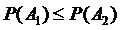(P5)  For any, we have(P6)  If,, then(P7)  For any, we have(P8)  For any, we have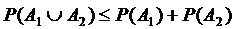(P9)  If, then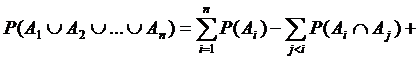This property is also called the inclusion-exclusion principle.

(P10)  Letbe events, with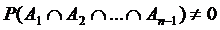. Then: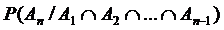The above properties represent formulas currently used in probability calculus on a finite field of events. Property (P9) is the main calculus formula for applications in finite cases.

Properties of probability on a σ-field

In addition, if  {Ω, Σ, P} is a σ-field, we also have the following properties:
(P11)   For any sequence of events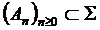with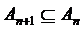(descending), we have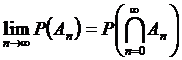. For any sequence of eventswith(ascending), we have.

(P12)   For any sequence of events, we have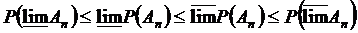.

(P13)   If the sequence of eventsis convergent (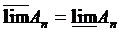), then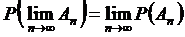.

(P14)   Generally,. The equality holds only if and only if the events are mutually exclusive.

Independent events. Conditional probability

Let us consider the experiment of tossing two coins and let Aheads on first coin and Bheads on second coin be two events. The occurrence of event A and its probability do not depend on the occurrence of event B, and vice versa. In this case, events A and B are said to be independent.

Definition:  Events A and B from the probability field {Ω, Σ, P} are said to be P-independent  if.

Example: In the previous example of the experiment of tossing two coins we have: P(A and B) = P(A) x P(B) = (1/2) x (1/2) = 1/4.

Consider an urn containing four white balls and three black balls. Two people extract one ball each from the urn. Let Afirst person is extracting a white ball and Bsecond person is extracting a white ball be two events. The probability of event B, in the absence of information about A, is 4/7. If event A has occurred, the probability of event B is 1/2, so event B depends on event A. Therefore, these two events are not independent. It is natural to call the probability of event B conditional on event A and to denote it by  P(BA).

Definition:  Let  {Ω, Σ, P} be a probability σ-field and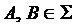with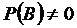. Call the probability of event A conditional on event B, the ratio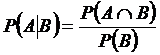.

Total probability formula. Bayes’s theorem
Definition: Call a complete system of events a finite or countable family of events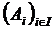, with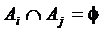for any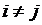,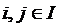and.

A complete system of events is therefore a partition of the sample space Ω.

Example: In the experiment of throwing a die, the system {1, 2, 3}, {4, 5}, {6} is a complete system of events, while {1, 2, 3}, {3, 4, 5}, {6} is not, as the first two events are not exclusive.

Theorem (the total probability formula):  Let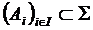be a complete system of events with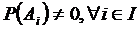. For any, we have.

Bayes’s Formula (the theorem of hypotheses):  Letbe a complete system of events. The probabilities of these events (hypotheses) are given before performing an experiment. The experiment produces another event A. Then,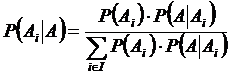, for every.are called marginal probabilities andandare called conditional probabilities.

Bayes’s formula shows how the probabilities of the hypotheses change with the occurrence of event A. Bayes’s theorem is a main result in probability theory, which relates the conditional and marginal probability of two aleatory events A and B. In some interpretations of probability, Bayes’s theorem explains how to update or revise beliefs in light of new evidence.

 Sources All properties of probability, the main results and theorems, including the random variables and classical probability distributions, along with suggestive examples and applications, are all exposed in a comprehensible manner in the book UNDERSTANDING AND CALCULATING THE ODDS: Probability Theory Basics and Calculus Guide for Beginners, with Applications in Games of Chance and Everyday Life.  You may find it in the Books section with a free sample.